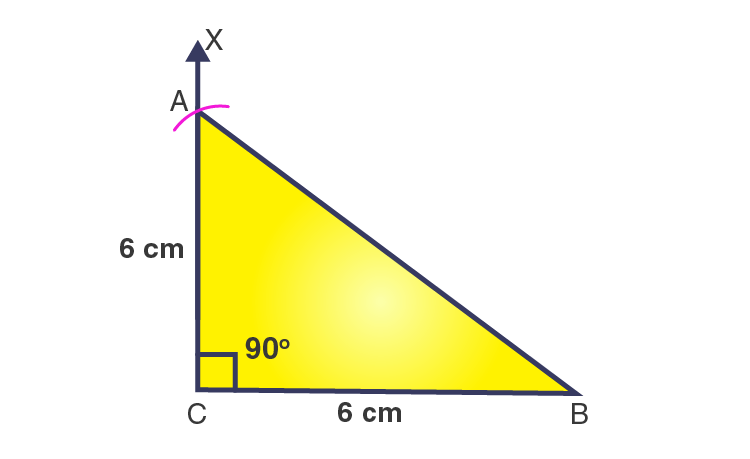# Construct an isosceles right-angled triangle ABC, where m∠ACB = 90° and AC = 6 cm.

SolutionThe steps for construction are given below:

1. Draw a line segment BC = 6 cm.

2. From point C, draw a ray CX to making an angle of 90o i.e. ∠XCB = 90o.

3. Considering C as a center and radius 6 cm, draw an arc that cuts the ray CX at A.

4. Join AB.

Then, ΔABC obtained is the required right angled triangle.(0)(0)